Next: Various Confusing Issues on Up: Lecture_26_web Previous: Lecture_26_web

The Gibbs Phase Rule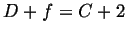(26-1)

The Gibbs phase rule is a very useful equation because it put precise limits on the number of phases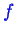that can be simultaneously in equilibrium for a given number of components.

What does Equation 26-1 mean? Consider the following example of a single component (pure) phase diagram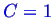.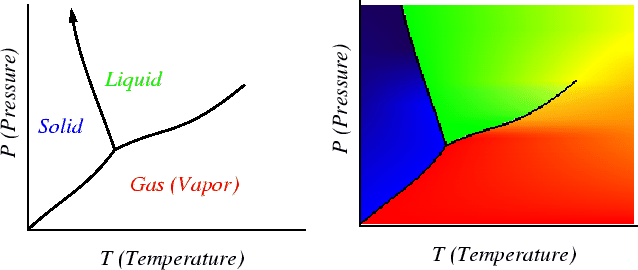Consider a single-phase region: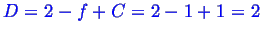This implies that two variables (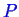and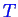) can be changed independently (i.e., pick any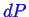and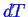) and a single phase remains in equilibrium.

Consider where two phases are in equilibrium: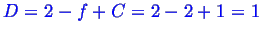,
There is only one degree of freedom-for the two phases to remain in equilibrium, one variable can be changed freely (for instance,) but then the change in the other variable (i.e.,) must depend on the change of the free variables: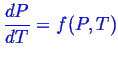Finally, consider where three phases are in equilibrium then: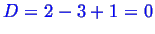.

There can be no change any variable that maintains three phase equilibrium.Next: Various Confusing Issues on Up: Lecture_26_web Previous: Lecture_26_web
W. Craig Carter 2002-11-21